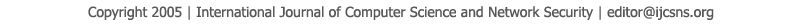To search, Click below search items.All Published Papers Search Service
 Title Author Year Keyword
 Title Comparing Different Estimators for Parameters of Two Gamma Parameters Using Simulation Author Mohmod M. Sh. Amrir Citation Vol. 14  No. 10  pp. 111-116 Abstract This paper deals with estimating two parameters of Gamma distribution, which are the shape parameter ( ), and the scale parameter ( ) using the method of moments, maximum likelihood, the scale parameter ( ) which is important in estimation of mean of life to failure distribution, are also estimated using Bayes estimator where ( ) considered random variable have prior distribution [ ] and proposed prior [ ] under squared error loss function. The third proposed estimator is ( ) which is the mixture of maximum likelihood ( ) and ( ) which is the first Bayes estimator, the value of proportion (p) which minimize the mean square error of ( ) is also derived. The comparison has been done through simulation using different sets of initial values and different sample size using mean square error (MSE). All results of comparison explained in tables Keywords Two Parameter Gamma URL http://paper.ijcsns.org/07_book/201410/20141017.pdf